# Azimuthal Quantum Number

## Quantum Number - Types of Quantum Numbers

For describing the unique quantum state of an electron in an atom, we use four types of quantum numbers-

• • Principle quantum number

• • Azimuthal quantum number

• • Magnetic quantum number

• • Spin quantum number

• In an atom, let's take a single electron. An electron is located on a shell which is divided into sub-shells and further divided into orbitals.

Principle quantum number directly describes the size and the energy level of the orbital. It is denoted by n. The value of n is always an integer. The principal quantum number tells us in which shell the electron is present on and it also identifies the number of sub-shells present.

The values n can take are n= 0,1,2,3, 4,

The shells have specific denotations.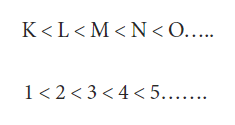Increase in n value leads to an increase in the size of the shell. As the size increases, the distance between the nucleus and electrons increases. This further leads to a decrease in the energy required to pull an electron out of the atom.

Therefore, n is directly proportional to the size and inversely proportional to the energy.

Azimuthally quantum number gives the sub-shell. It determines the angular momentum and the shape of an orbital. It can also be called orbital angular momentum quantum number or orbital quantum number or second quantum number. It is denoted by ℓ (pronounced ell).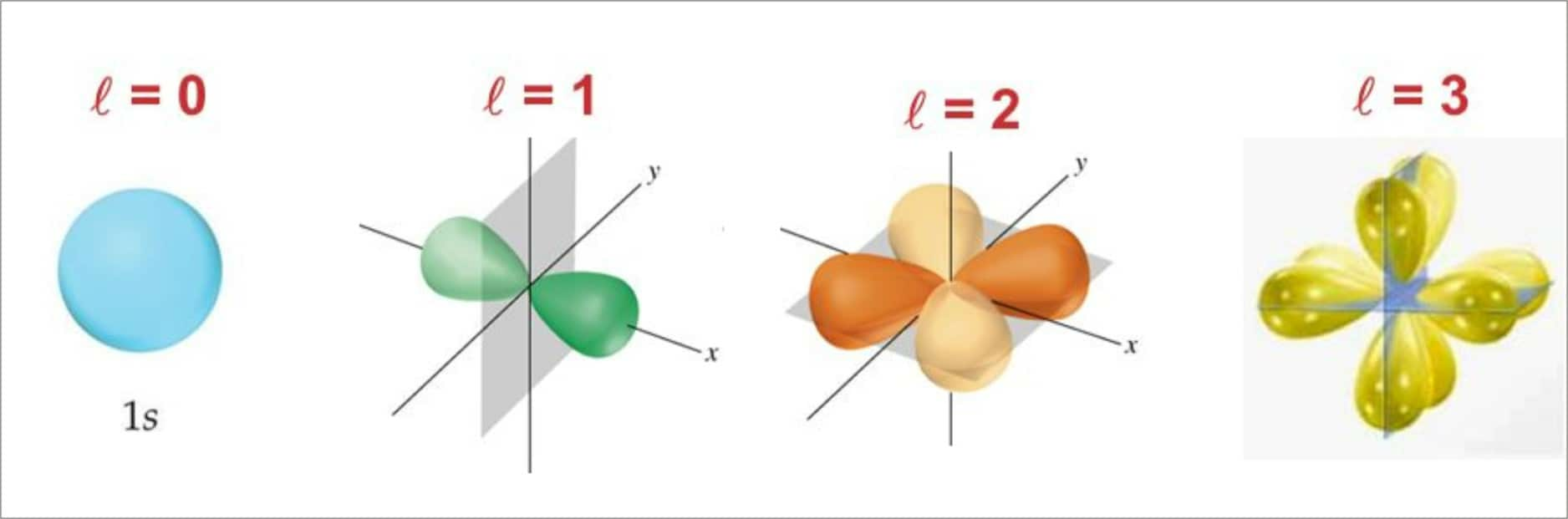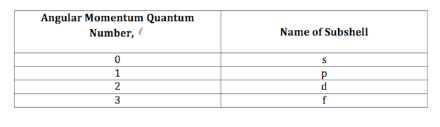RELATION BETWEEN n AND ℓ

The values of ℓ can vary from 0 to n-1

For example, if n=1 then ℓ can have the value as 0,

If n=2 then ℓ can have values as 0 and 1, and so on.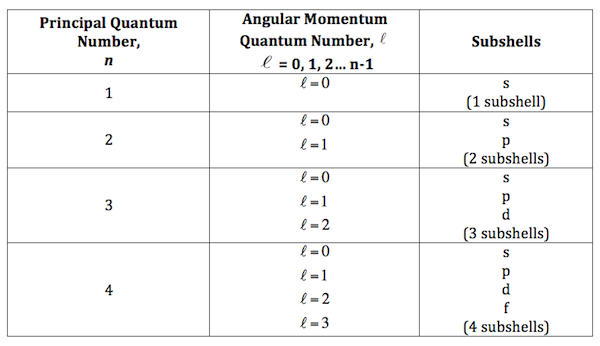THE S ORBITALS

The s orbital is symmetrically spherical in shape. The values for the azimuthal quantum number and magnetic quantum number are equal; the only variation possible is in the principle quantum number. The size increases with the increase in the n value and 2s are bigger than 1s, 3s bigger than 2s and so on.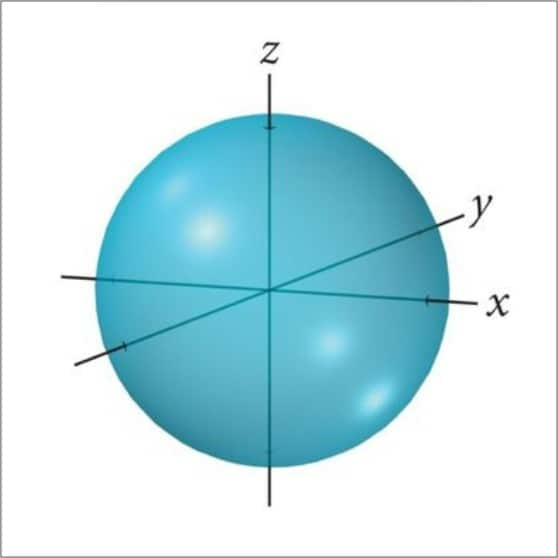THE P ORBITALS

Not all electrons inhabit the s orbital. The next orbital is p. The shape of this orbital is like two similar balloons attached at the nucleus, or it is called dumbbell shaped orbital. The p shell is made up of three orbitals pointing to three mutually perpendicular directions and they are arbitrarily given names as px, py, and pz.

THE d AND f ORBITALS

In the d shell there are 5 orbitals and f shell there are 7 orbitals. Since each orbital has two electrons, it makes 10 d orbital electrons and 14 f orbital electrons. The five d orbitals are denoted as day, dyz, dzx, dx2-y2, dz2.The energies of all the five orbitals is equal. The shape of the first four is similar to each other but it is different from the fifth one i.e. dz2.

The magnetic orbital quantum number gives us the spatial orientation of the orbitals with respect to the already existing standard co-ordinate axes. The number of possible values is given by 2ℓ+1. For example, if ℓ=1 then ml can have 3 values such as -1, 0, +1.

The spin quantum number gives the spin of the electrons. This has only two values +1/2 and -1/2.

These four quantum numbers are used to give the location of an electron in an atom.

NODES

It is a region of zero probability of finding electrons in an orbital. It is also called nodal surfaces.

In general, any ns orbital will have (n-1) nodes this implies that the number of nodes increases with an increase in the principal quantum number.

Any np orbital the number of nodes is given by n-2.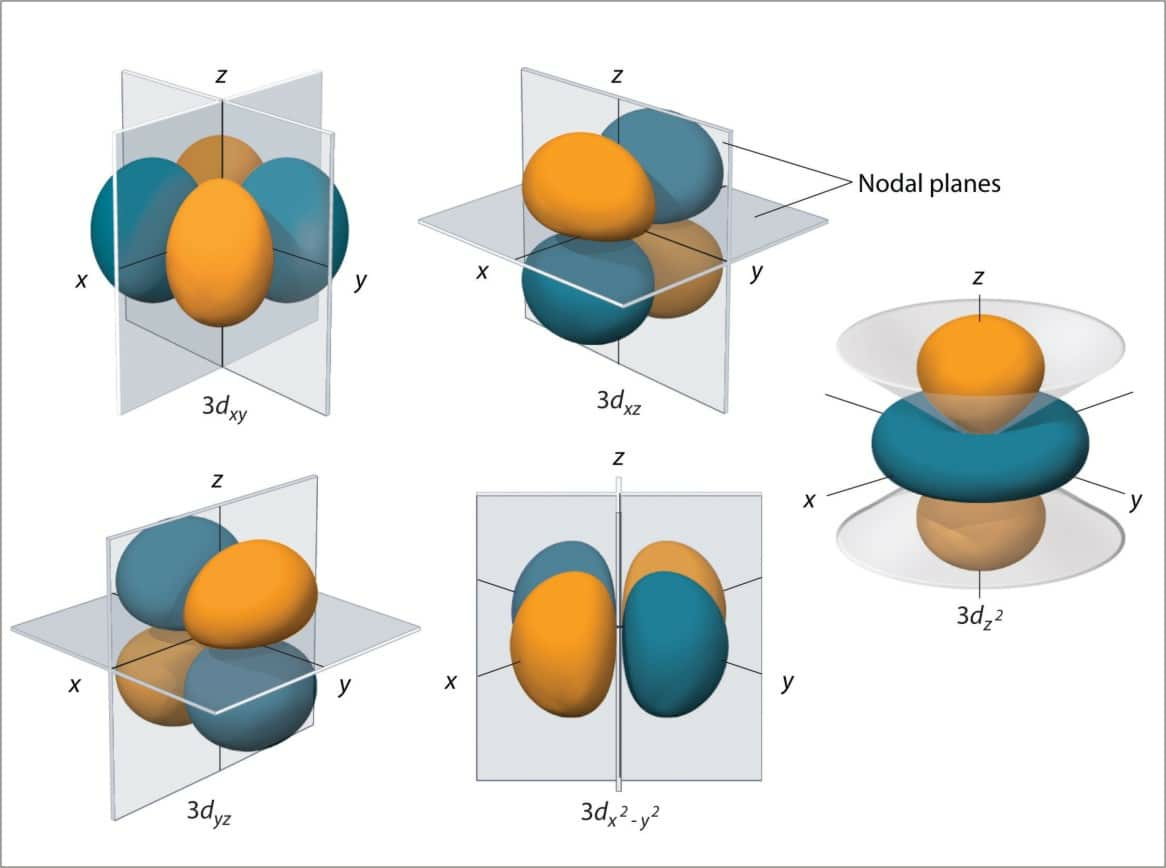BOUNDARY SURFACE DIAGRAMS

It is a diagram showing the probability density of finding an electron for different orbitals. This gives a fair representation of the shapes of the orbitals. The s orbital is said to be spherical which means that the probability of finding an electron in any direction at any given distance from the nucleus is equal within that sphere.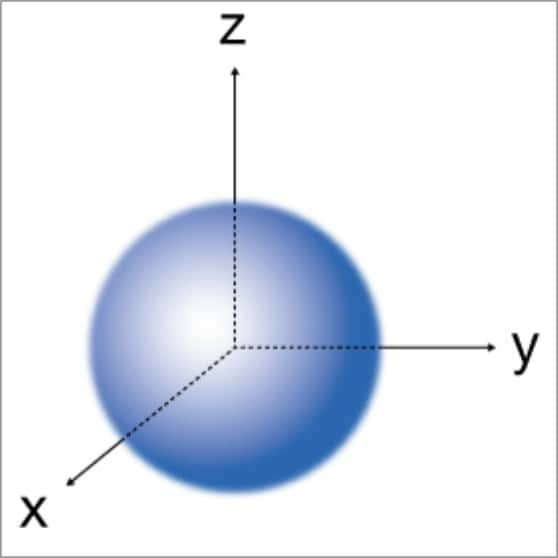ENERGIES OF THE ORBITALS

The energies of the orbitals solely depend on the principal quantum number. It goes like this 1s < 2s=2p < 3s = 3p = 3d< 4s =4p=4d< 5s=5p = 5d and so on. Even though the shape of 2s and 2p are different, but the energies of the electrons in these two orbitals are equal. The same is for 3s, 3p, and 3d. The different orbitals with the same energies are called degenerate orbitals.

The 1s orbitals are stable and are therefore called the ground state. The electrons when getting energy get an excited jump to higher energy orbitals. Then these orbitals are said to be in the excited state.

FILLING UP OF ORBITALS IN AN ATOM

The filling up of the orbitals in an atom takes place following three different principles-

• • Aufbau’s principle

• • Pauli exclusion principle

• • Hund’s rule of maximum multiplicity

• The Aufbau’s principle states that- in the ground state of the atoms, the orbitals are filled in order of their increasing energies. This means that the electron first fills up the orbital with the lowest energy and then move to orbitals with higher energy levels.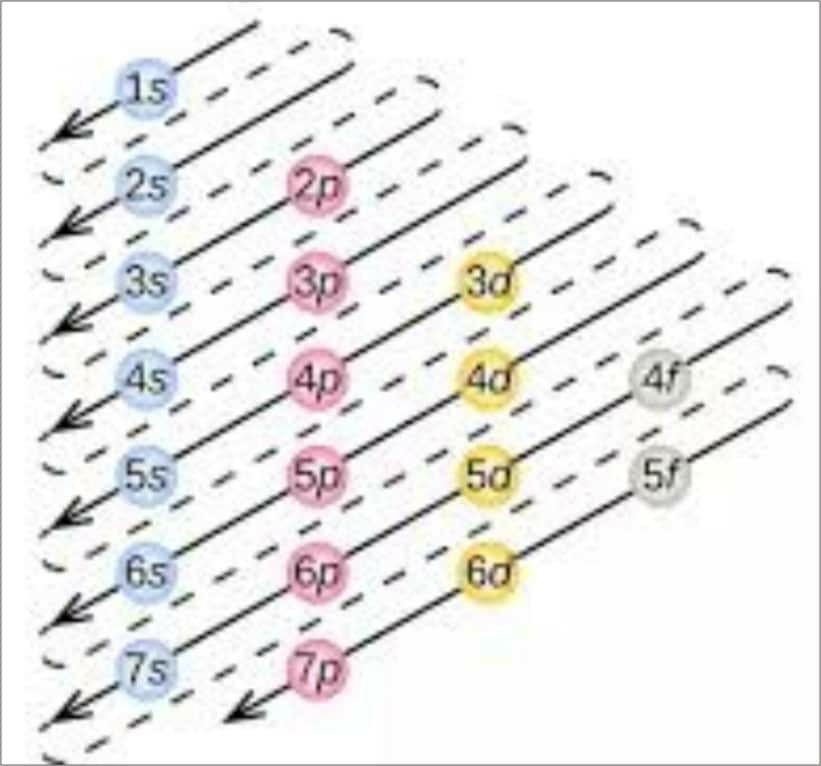Pauli Exclusion Principle states that- no two electrons can have the same set of four quantum numbers in an atom. This can also be stated as- only two electrons may exist in the same orbital and these electrons must have opposite spin. This means that even if the first three quantum numbers are the same the spin quantum number will be opposite for two electrons in an atom.

We know that in each orbital there are two electrons. Hund’s rule of maximum multiplicity is The basic principle by which filling up of these two places is given. It states that- pairing of electrons belonging to the same subshell in the orbitals (p, d or f) does not take place until each orbital belonging to that subshell is singly occupied (has got one electron each). This means that the second electron, for example, 3s will not fill up till 3d is not singly occupied. We know that p, d, and f respectively have 3, 5, and 7 orbitals. So, in these, the pairing of electrons will begin only from the 4th, 6th, and 8th electrons.
We, thus, come to the conclusion that we need all the four quantum numbers and the three principles to know how and which electrons will be located in an atom.